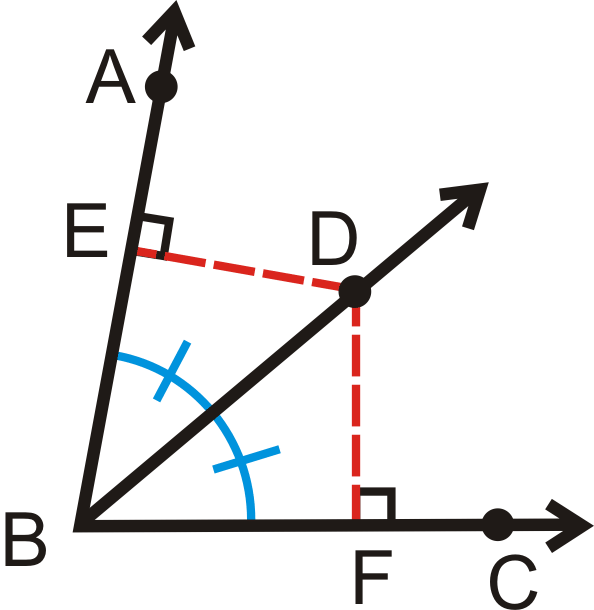Mahesh Godavarti
0

This is a great question, especially because you don't really get an answer in Google search directly with this phrase.

We have to put together the reason why the center of the inscribed circle is the intersection of the angle bisectors.

First, we will understand the property of angle bisectors, called the Angle Bisector Theorem, which states that every point on the angle bisector is equidistant from the two sides of the angle (i.e. ED = DF in the figure below). Conversely, if a point is equidistant from the two sides then it lies on the angle bisector of the angle made by the two sides.Second, we will understand that angle bisectors of the three angles in a triangle are concurrent, i.e. they all intersect at the same point.

This follows directly from the angle bisector theorem. A more formal proof can be found in the following link.

https://www.algebra.com/algebra/homework/Triangles/Angle-bisectors-of-a-triangle-are-concurrent.lesson

This essentially means that the point where angle bisectors intersect is equidistant from all three sides of a triangle, and is therefore, the center of the inscribed circle.